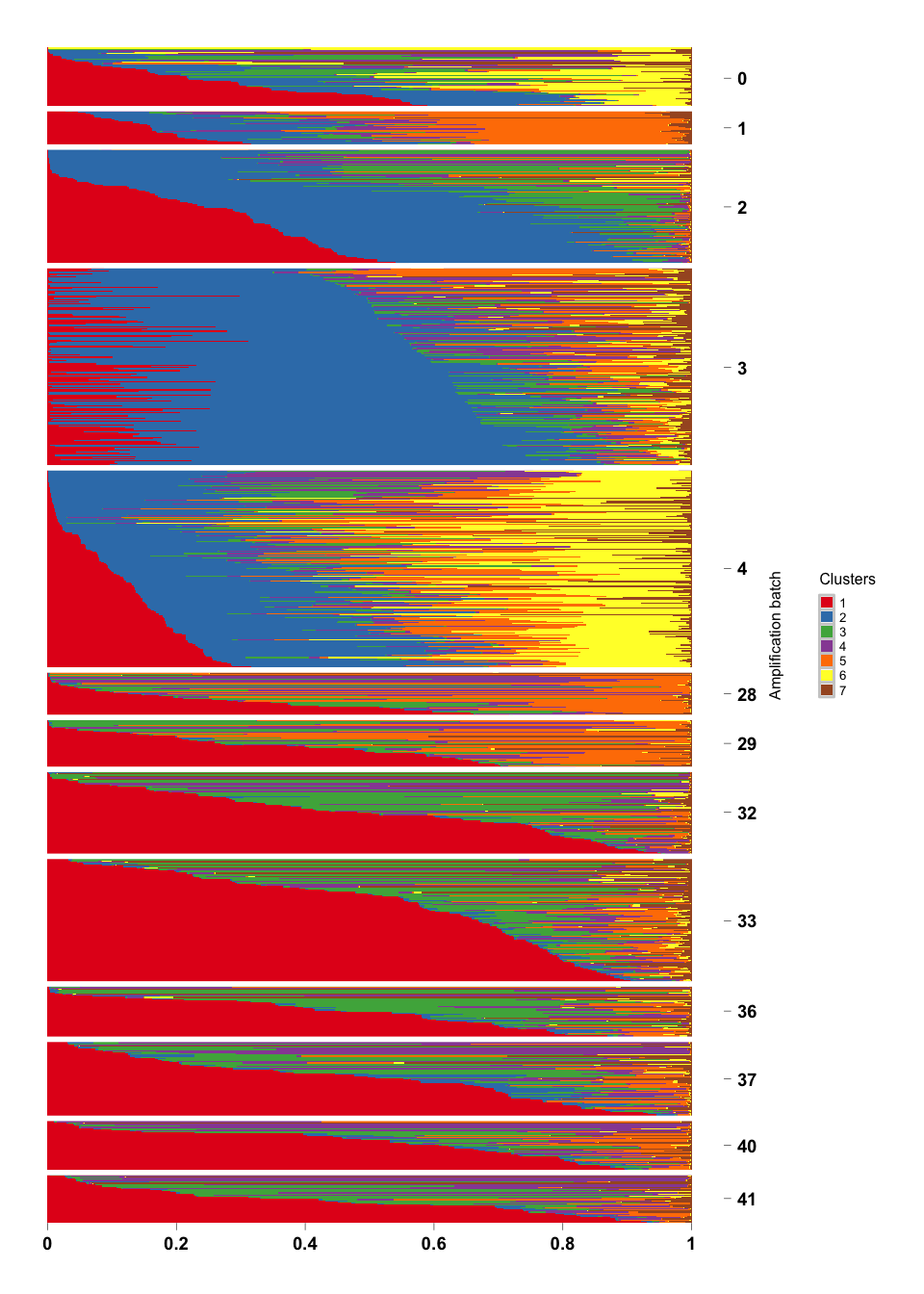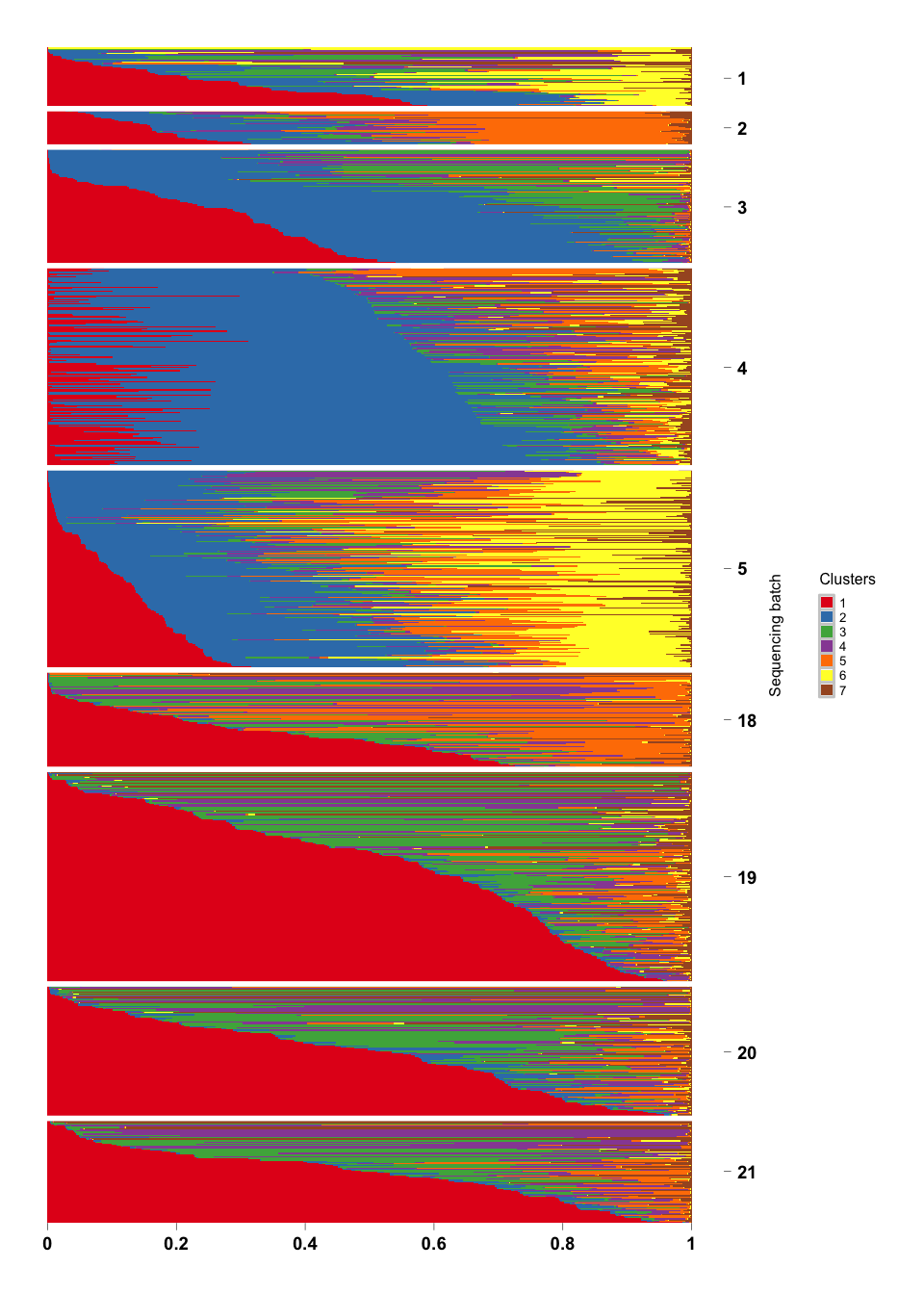## Objective

We apply the topic model for clustering of single cells in the data due to Jaitin . Check the paper.

## Load pacakges

library(CountClust)
library(Biobase)

## Data Preprocessing

# import raw data
#devtools::install_github('jhsiao999/singleCellRNASeqMouseJaitinSpleen')
library(singleCellRNASeqMouseJaitinSpleen)
data(MouseJaitinSpleen)
counts <- exprs(MouseJaitinSpleen)
gene_names <- rownames(fData(MouseJaitinSpleen))
meta_data <- pData(MouseJaitinSpleen)
dim(counts)
##  20190  4590
# exclude ERCC genes
ENSG_genes_index <- grep("ERCC", gene_names, invert = TRUE)
length(ENSG_genes_index)
##  20107
# expression matrix without ENSG genes
counts_ensg <- counts[ENSG_genes_index, ]

head(meta_data)
##    index sequencing_batch amplification_batch mouse_ID pool_barcode
## 7      1                1                   0     <NA>       NNNNNN
## 8      2                1                   0     <NA>       NNNNNN
## 9      3                1                   0     <NA>       NNNNNN
## 10     4                1                   0     <NA>       NNNNNN
## 11     5                1                   0     <NA>       NNNNNN
## 12     6                1                   0     <NA>       NNNNNN
##    sample_barcode plate_id well_id number_of_cells sorting_markers
## 7          CTACCA        1      A1               1          CD11c+
## 8          CATGCT        1      B1               1          CD11c+
## 9          GCACAT        1      C1               1          CD11c+
## 10         TGCTCG        1      D1               1          CD11c+
## 11         AGCAAT        1      E1               1          CD11c+
## 12         AGTTGC        1      F1               1          CD11c+
##    RMT_length group_name ERCC_dilution ERCC_volume_ul
## 7           4     CD11c+      2.00E-05           0.01
## 8           4     CD11c+      2.00E-05           0.01
## 9           4     CD11c+      2.00E-05           0.01
## 10          4     CD11c+      2.00E-05           0.01
## 11          4     CD11c+      2.00E-05           0.01
## 12          4     CD11c+      2.00E-05           0.01
##    Column_name_in_processed_data_file
## 7                                 0_1
## 8                                 0_2
## 9                                 0_3
## 10                                0_4
## 11                                0_5
## 12                                0_6
# exclude wells more than 1 cell and with total UMI count > 600
# also include only CD11c+ cells
sum(as.numeric(meta_data$number_of_cells) ==1 & meta_data$group_name =="CD11c+" & colSums(counts_ensg) > 600 )
##  1135
## exclude ribosomal protein genes and ...
filter_genes <- c("M34473","abParts","M13680","Tmsb4x",
"S100a4","B2m","Atpase6","Rpl23","Rps18",
"Rpl13","Rps19","H2-Ab1","Rplp1","Rpl4",
"Rps26","EF437368")
fcounts <- counts_ensg[ -match(filter_genes, rownames(counts_ensg)), ]
sample_counts <- colSums(fcounts)
sum(meta_data$number_of_cells==1 & meta_data$group_name=="CD11c+" &
sample_counts > 600 )
##  1041
## create sample filtering index
filter_sample_index <- which(meta_data$number_of_cells == 1 & meta_data$group_name == "CD11c+" &
sample_counts > 600)

## create a filtered dataset
counts_ensg_filtered <- counts_ensg[ , filter_sample_index]
meta_data_filtered <- meta_data[filter_sample_index, ]

## Topic model fit

We fit the topic on the filtered dataset (see above) for $$7$$ topics.

# transpose the count matrix from gene by sample to
# sample by gene, the default format of CountClust packge
# also the meta data matrix from sample by phenotpye to
# phenotype by sample
Topic_Clus <- StructureObj(data = t(counts_ensg_filtered),
samp_metadata = t(data.frame(meta_data_filtered)),
nclus_vec = 7,
plot = FALSE,
tol = 0.01,
path_rds = "rdas/MouseJaitinSpleen-topicFit.rds")

## Structure Plot (Amplification batch)

topic_fit <- readRDS("../rdas/MouseJaitinSpleen-topicFit.rds")

# extract the omega matrix: membership weights of each cell
names(topic_fit$clust_7) ##  "K" "theta" "omega" "BF" "D" "X" omega <- topic_fit$clust_7$omega filter_sample_index <- which(meta_data$number_of_cells == 1 &
meta_data\$group_name == "CD11c+" &
sample_counts > 600)

# make filterd phenotype data
meta_data_filtered <- meta_data[filter_sample_index, ]
stopifnot(dim(meta_data_filtered) == dim(omega))

# making annotation matrix
amp_batch <- as.numeric(meta_data_filtered[ , "amplification_batch"])
annotation <- data.frame(
sample_id = paste0("X", c(1:NROW(omega)) ),
tissue_label = factor(amp_batch,
levels = rev(sort(unique(amp_batch))) ) )
StructureGGplot(omega = omega,
annotation = annotation,
palette = RColorBrewer::brewer.pal(9, "Set1"),
yaxis_label = "Amplification batch",
axis_tick = list(axis_ticks_length = .1,
axis_ticks_lwd_y = .1,
axis_ticks_lwd_x = .1,
axis_label_size = 7,
axis_label_face = "bold"),
order_sample = TRUE)## Structure Plot (Sequencing Batch)

seq_batch <- as.numeric(meta_data_filtered[ , "sequencing_batch"])
annotation <- data.frame(
sample_id = paste0("X", c(1:NROW(omega)) ),
tissue_label = factor(seq_batch,
levels = rev(sort(unique(seq_batch))) ) )

StructureGGplot(omega = omega,
annotation = annotation,
palette = RColorBrewer::brewer.pal(9, "Set1"),
yaxis_label = "Sequencing batch",
axis_tick = list(axis_ticks_length = .1,
axis_ticks_lwd_y = .1,
axis_ticks_lwd_x = .1,
axis_label_size = 7,
axis_label_face = "bold"),
order_sample = TRUE)